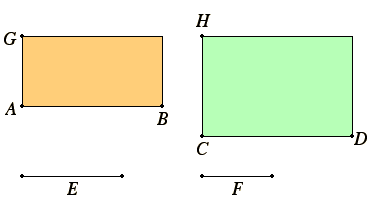# Proposition 16

If four straight lines are proportional, then the rectangle contained by the extremes equals the rectangle contained by the means; and, if the rectangle contained by the extremes equals the rectangle contained by the means, then the four straight lines are proportional.

Let the four straight lines AB, CD, E, and F be proportional, so that AB is to CD as E is to F.

I say that the rectangle AB by F equals the rectangle CD by E.

Draw AG and CH from the points A and C at right angles to the straight lines AB and CD, and make AG equal to F, and CH equal to E.

I.31

Complete the parallelograms BG and DH.V.7

Then since AB is to CD as E is to F, while E equals CH, and F equals AG, therefore AB is to CD as CH is to AG.

Therefore in the parallelograms BG and DH the sides about the equal angles are reciprocally proportional.

VI.14

But those equiangular parallelograms in which the sides about the equal angles are reciprocally proportional are equal, therefore the parallelogram BG equals the parallelogram DH.

And BG is the rectangle AB by F, for AG equals F, and DH is the rectangle CD by E, for E equals CH, therefore the rectangle AB by F equals the rectangle CD by E.

Next, let the rectangle AB by F be equal to the rectangle CD by E.

I say that the four straight lines are proportional, so that AB is to CD as E is to F.

With the same construction, since the rectangle AB by F equals the rectangle CD by E, and the rectangle AB by F is BG, for AG equals F, and the rectangle CD by E is DH, for CH equals E, therefore BG equals DH.

VI.14

And they are equiangular. But in equal and equiangular parallelograms the sides about the equal angles are reciprocally proportional.

V.7

Therefore AB is to CD as CH is to AG.

But CH equals E, and AG to F, therefore AB is to CD as E is to F.

Therefore, if four straight lines are proportional, then the rectangle contained by the extremes equals the rectangle contained by the means; and, if the rectangle contained by the extremes equals the rectangle contained by the means, then the four straight lines are proportional.

Q.E.D.

## Guide

This proposition is a special case of VI.14. It hardly needs such a protracted proof. It is used occasionally in Book X, but the special case when the means are equal and the second figure is a square, as enunciated in the next proposition, is used throughout Book X and frequently in Book XIII.